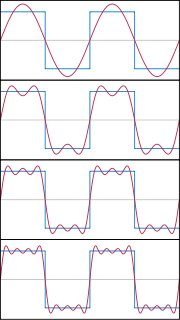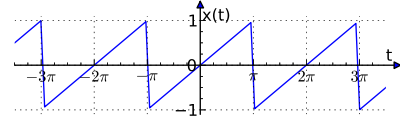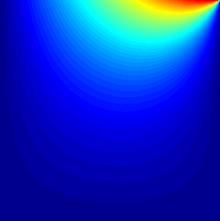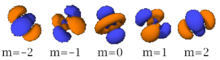# Fourier series

﻿
Fourier seriesThe first four Fourier series approximations for a square wave.

In mathematics, a Fourier series decomposes periodic functions or periodic signals into the sum of a (possibly infinite) set of simple oscillating functions, namely sines and cosines (or complex exponentials). The study of Fourier series is a branch of Fourier analysis. Fourier series were introduced by Joseph Fourier (1768–1830) for the purpose of solving the heat equation in a metal plate.

The heat equation is a partial differential equation. Prior to Fourier's work, no solution to the heat equation was known in the general case, although particular solutions were known if the heat source behaved in a simple way, in particular, if the heat source was a sine or cosine wave. These simple solutions are now sometimes called eigensolutions. Fourier's idea was to model a complicated heat source as a superposition (or linear combination) of simple sine and cosine waves, and to write the solution as a superposition of the corresponding eigensolutions. This superposition or linear combination is called the Fourier series.

Although the original motivation was to solve the heat equation, it later became obvious that the same techniques could be applied to a wide array of mathematical and physical problems, and especially those involving linear differential equations with constant coefficients, for which the eigensolutions are sinusoids. The Fourier series has many such applications in electrical engineering, vibration analysis, acoustics, optics, signal processing, image processing, quantum mechanics, econometrics, thin-walled shell theory, etc.

The Fourier series is named in honour of Joseph Fourier (1768–1830), who made important contributions to the study of trigonometric series, after preliminary investigations by Leonhard Euler, Jean le Rond d'Alembert, and Daniel Bernoulli. He applied this technique to find the solution of the heat equation, publishing his initial results in his 1807 Mémoire sur la propagation de la chaleur dans les corps solides (trans. Treatise on the propagation of heat in solid bodies), and publishing his Théorie analytique de la chaleur in 1822.

From a modern point of view, Fourier's results are somewhat informal, due to the lack of a precise notion of function and integral in the early nineteenth century. Later, Dirichlet and Riemann expressed Fourier's results with greater precision and formality.

## Revolutionary article

 “$\varphi(y)=a\cos\frac{\pi y}{2}+a'\cos 3\frac{\pi y}{2}+a''\cos5\frac{\pi y}{2}+\cdots.$ Multiplying both sides by$\cos(2k+1)\frac{\pi y}{2}$, and then integrating from y = − 1 to y = + 1 yields:$a_k=\int_{-1}^1\varphi(y)\cos(2k+1)\frac{\pi y}{2}\,dy.$ ” —Joseph Fourier, Mémoire sur la propagation de la chaleur dans les corps solides. (1807)[nb 1]

This immediately gives any coefficient ak of the trigonometrical series for φ(y) for any function which has such an expansion. It works because if φ has such an expansion, then (under suitable convergence assumptions) the integral\begin{align} a_k&=\int_{-1}^1\varphi(y)\cos(2k+1)\frac{\pi y}{2}\,dy \\ &= \int_{-1}^1\left(a\cos\frac{\pi y}{2}\cos(2k+1)\frac{\pi y}{2}+a'\cos 3\frac{\pi y}{2}\cos(2k+1)\frac{\pi y}{2}+\cdots\right)\,dy \end{align}

can be carried out term-by-term. But all terms involving$\cos(2j+1)\frac{\pi y}{2} \cos(2k+1)\frac{\pi y}{2}$ for jk vanish when integrated from −1 to 1, leaving only the kth term.

In these few lines, which are close to the modern formalism used in Fourier series, Fourier revolutionized both mathematics and physics. Although similar trigonometric series were previously used by Euler, d'Alembert, Daniel Bernoulli and Gauss, Fourier believed that such trigonometric series could represent arbitrary functions. In what sense that is actually true is a somewhat subtle issue and the attempts over many years to clarify this idea have led to important discoveries in the theories of convergence, function spaces, and harmonic analysis.

When Fourier submitted a later competition essay in 1811, the committee (which included Lagrange, Laplace, Malus and Legendre, among others) concluded: ...the manner in which the author arrives at these equations is not exempt of difficulties and...his analysis to integrate them still leaves something to be desired on the score of generality and even rigour.

## Birth of harmonic analysis

Since Fourier's time, many different approaches to defining and understanding the concept of Fourier series have been discovered, all of which are consistent with one another, but each of which emphasizes different aspects of the topic. Some of the more powerful and elegant approaches are based on mathematical ideas and tools that were not available at the time Fourier completed his original work. Fourier originally defined the Fourier series for real-valued functions of real arguments, and using the sine and cosine functions as the basis set for the decomposition.

Many other Fourier-related transforms have since been defined, extending the initial idea to other applications. This general area of inquiry is now sometimes called harmonic analysis. A Fourier series, however, can be used only for periodic functions, or for functions on a bounded (compact) interval.

## Definition

In this section, ƒ(x) denotes a function of the real variable x. This function is usually taken to be periodic, of period 2π, which is to say that ƒ(x + 2π) = ƒ(x), for all real numbers x. We will attempt to write such a function as an infinite sum, or series of simpler 2π–periodic functions. We will start by using an infinite sum of sine and cosine functions on the interval [−ππ], as Fourier did (see the quote above), and we will then discuss different formulations and generalizations.

### Fourier's formula for 2π-periodic functions using sines and cosines

For a periodic function ƒ(x) that is integrable on [−ππ], the numbers$a_n = \frac{1}{\pi}\int_{-\pi}^\pi f(x) \cos(nx)\, dx, \quad n \ge 0$

and$b_n = \frac{1}{\pi}\int_{-\pi}^\pi f(x) \sin(nx)\, dx, \quad n \ge 1$

are called the Fourier coefficients of ƒ. One introduces the partial sums of the Fourier series for ƒ, often denoted by$(S_N f)(x) = \frac{a_0}{2} + \sum_{n=1}^N \, [a_n \cos(nx) + b_n \sin(nx)], \quad N \ge 0.$

The partial sums for ƒ are trigonometric polynomials. One expects that the functions SN ƒ approximate the function ƒ, and that the approximation improves as N tends to infinity. The infinite sum$\frac{a_0}{2} + \sum_{n=1}^\infty \, [a_n \cos(nx) + b_n \sin(nx)]$

is called the Fourier series of ƒ. These trigonometric functions can themselves be expanded, using multiple angle formulae.

The Fourier series does not always converge, and even when it does converge for a specific value x0 of x, the sum of the series at x0 may differ from the value ƒ(x0) of the function. It is one of the main questions in harmonic analysis to decide when Fourier series converge, and when the sum is equal to the original function. If a function is square-integrable on the interval [−ππ], then the Fourier series converges to the function at almost every point. In engineering applications, the Fourier series is generally presumed to converge everywhere except at discontinuities, since the functions encountered in engineering are more well behaved than the ones that mathematicians can provide as counter-examples to this presumption. In particular, the Fourier series converges absolutely and uniformly to ƒ(x) whenever the derivative of ƒ(x) (which may not exist everywhere) is square integrable. See Convergence of Fourier series.

It is possible to define Fourier coefficients for more general functions or distributions, in such cases convergence in norm or weak convergence is usually of interest.

#### Example 1: a simple Fourier seriesPlot of a periodic identity function—a sawtooth wave.Animated plot of the first five successive partial Fourier series.

We now use the formula above to give a Fourier series expansion of a very simple function. Consider a sawtooth wave$f(x) = x, \quad \mathrm{for } -\pi < x < \pi,$$f(x + 2\pi) = f(x), \quad \mathrm{for } -\infty < x < \infty.$

In this case, the Fourier coefficients are given by\begin{align} a_0 &{} = \frac{1}{\pi}\int_{-\pi}^{\pi}x\,dx = 0. \\ a_n &{} = \frac{1}{\pi}\int_{-\pi}^{\pi}x \cos(nx)\,dx = 0, \quad n \ge 0. \\ b_n &{}= \frac{1}{\pi}\int_{-\pi}^{\pi} x \sin(nx)\, dx = -\frac{2}{n}\cos(n\pi) + \frac{2}{\pi n^2}\sin(n\pi) = 2 \, \frac{(-1)^{n+1}}{n}, \quad n \ge 1.\end{align}

It can be proven that the Fourier series converges to ƒ(x) at every point x where ƒ is differentiable, and therefore:\begin{align} f(x) &= \frac{a_0}{2} + \sum_{n=1}^\infty \left[a_n\cos\left(nx\right)+b_n\sin\left(nx\right)\right] \\ &=2\sum_{n=1}^\infty \frac{(-1)^{n+1}}{n} \sin(nx), \quad \mathrm{for} \quad x - \pi \notin 2 \pi \mathbf{Z}. \end{align}

(Eq.1)

When x = π, the Fourier series converges to 0, which is the half-sum of the left- and right-limit of ƒ at x = π. This is a particular instance of the Dirichlet theorem for Fourier series.

#### Example 2: Fourier's motivationHeat distribution in a metal plate, using Fourier's method

One notices that the Fourier series expansion of our function in example 1 looks much less simple than the formula ƒ(x) = x, and so it is not immediately apparent why one would need this Fourier series. While there are many applications, we cite Fourier's motivation of solving the heat equation. For example, consider a metal plate in the shape of a square whose side measures π meters, with coordinates (xy) ∈ [0, π] × [0, π]. If there is no heat source within the plate, and if three of the four sides are held at 0 degrees Celsius, while the fourth side, given by y = π, is maintained at the temperature gradient T(xπ) = x degrees Celsius, for x in (0, π), then one can show that the stationary heat distribution (or the heat distribution after a long period of time has elapsed) is given by$T(x,y) = 2\sum_{n=1}^\infty \frac{(-1)^{n+1}}{n} \sin(nx) {\sinh(ny) \over \sinh(n\pi)}.$

Here, sinh is the hyperbolic sine function. This solution of the heat equation is obtained by multiplying each term of  Eq.1 by sinh(ny)/sinh(nπ). While our example function f(x) seems to have a needlessly complicated Fourier series, the heat distribution T(xy) is nontrivial. The function T cannot be written as a closed-form expression. This method of solving the heat problem was made possible by Fourier's work.

#### Other applications

Another application of this Fourier series is to solve the Basel problem by using Parseval's theorem. The example generalizes and one may compute ζ(2n), for any positive integer n.

### Exponential Fourier series

We can use Euler's formula,$e^{inx} = \cos(nx)+i\sin(nx), \,$

where i is the imaginary unit, to give a more concise formula:$f(x) = \sum_{n=-\infty}^ \infty c_n e^{inx}.$

The Fourier coefficients are then given by:$c_n = \frac{1}{2\pi}\int_{-\pi}^{\pi} f(x) e^{-inx}\, dx.$

The Fourier coefficients an, bn, cn are related via$a_n = { c_n + c_{-n} } \quad \text{ for }n=0,1,2,\dots\,$$b_n = i( c_{n} - c_{-n} ) \quad \text{ for }n=1,2,\dots\,$

and$c_n = \begin{cases} \frac{1}{2}(a_n - i b_n) & n > 0 \\ \quad \frac{1}{2}a_0 & n = 0 \\ \frac{1}{2}(a_{-n} + i b_{-n}) & n < 0 \end{cases}$

The notation cn is inadequate for discussing the Fourier coefficients of several different functions. Therefore it is customarily replaced by a modified form of ƒ (in this case), such as F or$\scriptstyle\hat{f},$  and functional notation often replaces subscripting.  Thus:\begin{align} f(x) &= \sum_{n=-\infty}^\infty \hat{f}(n)\cdot e^{inx} \\ &= \sum_{n=-\infty}^\infty F[n]\cdot e^{inx} \quad \text{(engineering)}. \end{align}

In engineering, particularly when the variable x represents time, the coefficient sequence is called a frequency domain representation. Square brackets are often used to emphasize that the domain of this function is a discrete set of frequencies.

### Fourier series on a general interval [a, a + τ]

The following formula, with appropriate complex-valued coefficients G[n], is a periodic function with period τ on all of R:$g(x)=\sum_{n=-\infty}^\infty G[n]\cdot e^{i 2\pi \frac{n}{\tau} x}.$

If a function is square-integrable in the interval [aa + τ], it can be represented in that interval by the formula above.  I.e., when the coefficients are derived from a function, h(x), as follows:$G[n] = \frac{1}{\tau}\int_a^{a+\tau} h(x)\cdot e^{-i 2\pi \frac{n}{\tau} x}\, dx,$

then g(x) will equal h(x) in the interval [a,a+τ ]. It follows that if h(x) is τ-periodic, then:

• g(x) and h(x) are equal everywhere, except possibly at discontinuities, and
• a is an arbitrary choice. Two popular choices are a = 0, and a = −τ/2.

Another commonly used frequency domain representation uses the Fourier series coefficients to modulate a Dirac comb:$G(f) \ \stackrel{\mathrm{def}}{=} \ \sum_{n=-\infty}^\infty G[n]\cdot \delta \left(f-\frac{n}{\tau}\right),$

where variable ƒ represents a continuous frequency domain. When variable x has units of seconds, ƒ has units of hertz. The "teeth" of the comb are spaced at multiples (i.e. harmonics) of 1/τ, which is called the fundamental frequency.  g(x) can be recovered from this representation by an inverse Fourier transform:\begin{align} \mathcal{F}^{-1}\{G(f)\} &= \int_{-\infty}^\infty \left( \sum_{n=-\infty}^\infty G[n]\cdot \delta \left(f-\frac{n}{\tau}\right)\right) e^{i 2 \pi f x}\,df, \\ &= \sum_{n=-\infty}^\infty G[n]\cdot \int_{-\infty}^\infty \delta\left(f-\frac{n}{\tau}\right) e^{i 2 \pi f x}\,df, \\ &= \sum_{n=-\infty}^\infty G[n]\cdot e^{i2\pi \frac{n}{\tau} x} \ \ \stackrel{\mathrm{def}}{=} \ g(x). \end{align}

The function G(ƒ) is therefore commonly referred to as a Fourier transform, even though the Fourier integral of a periodic function is not convergent at the harmonic frequencies.

### Fourier series on a square

We can also define the Fourier series for functions of two variables x and y in the square [−ππ]×[−ππ]:$f(x,y) = \sum_{j,k \in \mathbb{Z}\text{ (integers)}} c_{j,k}e^{ijx}e^{iky},$$c_{j,k} = {1 \over 4 \pi^2} \int_{-\pi}^\pi \int_{-\pi}^\pi f(x,y) e^{-ijx}e^{-iky}\, dx \, dy.$

Aside from being useful for solving partial differential equations such as the heat equation, one notable application of Fourier series on the square is in image compression. In particular, the jpeg image compression standard uses the two-dimensional discrete cosine transform, which is a Fourier transform using the cosine basis functions.

### Hilbert space interpretation

In the language of Hilbert spaces, the set of functions$\{ e_n = e^{i n x},n\in\mathbb{Z}\}$ is an orthonormal basis for the space L2([ − π,π]) of square-integrable functions of [ − π,π]. This space is actually a Hilbert space with an inner product given for any two elements f and g by:$\langle f,\, g \rangle \;\stackrel{\mathrm{def}}{=} \; \frac{1}{2\pi}\int_{-\pi}^{\pi} f(x)\overline{g(x)}\,dx.$

The basic Fourier series result for Hilbert spaces can be written as$f=\sum_{n=-\infty}^\infty \langle f,e_n \rangle \, e_n.$

This corresponds exactly to the complex exponential formulation given above. The version with sines and cosines is also justified with the Hilbert space interpretation. Indeed, the sines and cosines form an orthogonal set:$\int_{-\pi}^{\pi} \cos(mx)\, \cos(nx)\, dx = \pi \delta_{mn}, \quad m, n \ge 1, \,$$\int_{-\pi}^{\pi} \sin(mx)\, \sin(nx)\, dx = \pi \delta_{mn}, \quad m, n \ge 1$

(where δmn is the Kronecker delta), and$\int_{-\pi}^{\pi} \cos(mx)\, \sin(nx)\, dx = 0 \, ;\,$

furthermore, the sines and cosines are orthogonal to the constant function 1. An orthonormal basis for L2([−ππ]) consisting of real functions is formed by the functions 1, and √2 cos(n  x),  √2 sin(n x) for n = 1, 2,...  The density of their span is a consequence of the Stone–Weierstrass theorem, but follows also from the properties of classical kernels like the Fejér kernel.

## Properties

We say that ƒ belongs to$C^k(\mathbb{T})$  if ƒ is a 2π-periodic function on R which is k times differentiable, and its kth derivative is continuous.

• If ƒ is a 2π-periodic odd function, then an = 0  for all n.
• If ƒ is a 2π-periodic even function, then bn = 0  for all n.
• If ƒ is integrable,$\lim_{|n|\rightarrow \infty}\hat{f}(n)=0$,$\lim_{n\rightarrow +\infty}a_n=0$ and$\lim_{n\rightarrow +\infty}b_n=0.$ This result is known as the Riemann–Lebesgue lemma.
• A doubly infinite sequence {an} in$c_0(\mathbb{Z})$ is the sequence of Fourier coefficients of a function in L1[0,2π] if and only if it is a convolution of two sequences in$\ell^2(\mathbb{Z})$. See 
• If$f \in C^1(\mathbb{T})$, then the Fourier coefficients$\widehat{f'}(n)$ of the derivative f' can be expressed in terms of the Fourier coefficients$\hat{f}(n)$ of the function f, via the formula$\widehat{f'}(n) = in \hat{f}(n)$.
• If$f \in C^k(\mathbb{T})$, then$\widehat{f^{(k)}}(n) = (in)^k \hat{f}(n)$. In particular, since$\widehat{f^{(k)}}(n)$ tends to zero, we have that$|n|^k\hat{f}(n)$ tends to zero, which means that the Fourier coefficients converge to zero faster than the kth power of n.
• Parseval's theorem. If$f \in L^2([-\pi,\pi])$, then$\sum_{n=-\infty}^\infty |\hat{f}(n)|^2 = \frac{1}{2\pi}\int_{-\pi}^{\pi} |f(x)|^2 \, dx$.
• Plancherel's theorem. If$c_0,\, c_{\pm 1},\, c_{\pm 2},\ldots$ are coefficients and$\sum_{n=-\infty}^\infty |c_n|^2 < \infty$ then there is a unique function$f\in L^2([-\pi,\pi])$ such that$\hat{f}(n) = c_n$ for every n.
• The first convolution theorem states that if ƒ and g are in L1([−π, π]), then$\widehat{f*g}(n) = 2\pi\hat{f}(n)\hat{g}(n)$, where ƒ ∗ g denotes the 2π-periodic convolution of ƒ and g. (The factor is not necessary for 1-periodic functions.)
• The second convolution theorem states that$\widehat{f\cdot g} = \hat{f}*\hat{g}$.$\sum_{n=-\infty}^\infty f(t + nT) = \frac{1}{T} \sum_{k=-\infty}^\infty \hat{f}\left(\frac{k}{T}\right)\ \exp\left(i 2\pi \frac{k}{T} t\right)$.

Similarly, the periodic summation of$\hat{f}$ has a Fourier series representation whose coefficients are proportional to discrete samples of f, a fact which provides a pictorial understanding of aliasing and the famous sampling theorem.

### Compact groups

One of the interesting properties of the Fourier transform which we have mentioned, is that it carries convolutions to pointwise products. If that is the property which we seek to preserve, one can produce Fourier series on any compact group. Typical examples include those classical groups that are compact. This generalizes the Fourier transform to all spaces of the form L2(G), where G is a compact group, in such a way that the Fourier transform carries convolutions to pointwise products. The Fourier series exists and converges in similar ways to the [−ππ] case.

An alternative extension to compact groups is the Peter–Weyl theorem, which proves results about representations of compact groups analogous to those about finite groups.

### Riemannian manifoldsThe atomic orbitals of chemistry are spherical harmonics and can be used to produce Fourier series on the sphere.

If the domain is not a group, then there is no intrinsically defined convolution. However, if X is a compact Riemannian manifold, it has a Laplace–Beltrami operator. The Laplace–Beltrami operator is the differential operator that corresponds to Laplace operator for the Riemannian manifold X. Then, by analogy, one can consider heat equations on X. Since Fourier arrived at his basis by attempting to solve the heat equation, the natural generalization is to use the eigensolutions of the Laplace–Beltrami operator as a basis. This generalizes Fourier series to spaces of the type L2(X), where X is a Riemannian manifold. The Fourier series converges in ways similar to the [−ππ] case. A typical example is to take X to be the sphere with the usual metric, in which case the Fourier basis consists of spherical harmonics.

### Locally compact Abelian groups

The generalization to compact groups discussed above does not generalize to noncompact, nonabelian groups. However, there is a straightfoward generalization to Locally Compact Abelian (LCA) groups.

This generalizes the Fourier transform to L1(G) or L2(G), where G is an LCA group. If G is compact, one also obtains a Fourier series, which converges similarly to the [−ππ] case, but if G is noncompact, one obtains instead a Fourier integral. This generalization yields the usual Fourier transform when the underlying locally compact Abelian group is$\mathbb{R}$.

## Approximation and convergence of Fourier series

An important question for the theory as well as applications is that of convergence. In particular, it is often necessary in applications to replace the infinite series$\sum_{-\infty}^\infty$  by a finite one,$(S_N f)(x) = \sum_{n=-N}^N \hat{f}(n) e^{inx}.$

This is called a partial sum. We would like to know, in which sense does (SN ƒ)(x) converge to ƒ(x) as N tends to infinity.

### Least squares property

We say that p is a trigonometric polynomial of degree N when it is of the form$p(x)=\sum_{n=-N}^N p_n e^{inx}.$

Note that SN ƒ is a trigonometric polynomial of degree N. Parseval's theorem implies that

Theorem. The trigonometric polynomial SN ƒ is the unique best trigonometric polynomial of degree N approximating ƒ(x), in the sense that, for any trigonometric polynomial$p\neq S_N f$ of degree N, we have$\|S_N f - f\|_2 < \|p - f\|_2.$

Here, the Hilbert space norm is$\| g \|_2 = \sqrt{{1 \over 2\pi} \int_{-\pi}^{\pi} |g(x)|^2 \, dx}.$

### Convergence

Because of the least squares property, and because of the completeness of the Fourier basis, we obtain an elementary convergence result.

Theorem. If ƒ belongs to L2([−π, π]), then the Fourier series converges to ƒ in L2([−π, π]), that is,$\|S_N f - f\|_2$ converges to 0 as N goes to infinity.

We have already mentioned that if ƒ is continuously differentiable, then$i n \hat{f}(n)$  is the nth Fourier coefficient of the derivative ƒ′. It follows, essentially from the Cauchy–Schwarz inequality, that the Fourier series of ƒ is absolutely summable. The sum of this series is a continuous function, equal to ƒ, since the Fourier series converges in the mean to ƒ:

Theorem. If$f \in C^1(\mathbb{T})$, then the Fourier series converges to ƒ uniformly (and hence also pointwise.)

This result can be proven easily if ƒ is further assumed to be C2, since in that case$n^2\hat{f}(n)$ tends to zero as$n\to\infty$. More generally, the Fourier series is absolutely summable, thus converges uniformly to ƒ, provided that ƒ satisfies a Hölder condition of order α > ½. In the absolutely summable case, the inequality$\sup_x |f(x) - (S_N f)(x)| \le \sum_{|n| > N} |\hat{f}(n)|$  proves uniform convergence.

Many other results concerning the convergence of Fourier series are known, ranging from the moderately simple result that the series converges at x if ƒ is differentiable at x, to Lennart Carleson's much more sophisticated result that the Fourier series of an L2 function actually converges almost everywhere.

These theorems, and informal variations of them that don't specify the convergence conditions, are sometimes referred to generically as "Fourier's theorem" or "the Fourier theorem".

### Divergence

Since Fourier series have such good convergence properties, many are often surprised by some of the negative results. For example, the Fourier series of a continuous T-periodic function need not converge pointwise. The uniform boundedness principle yields a simple non-constructive proof of this fact.

In 1922, Andrey Kolmogorov published an article entitled "Une série de Fourier-Lebesgue divergente presque partout" in which he gave an example of a Lebesgue-integrable function whose Fourier series diverges almost everywhere. He later constructed an example of an integrable function whose Fourier series diverges everywhere (Katznelson 1976).

Wikimedia Foundation. 2010.

### Look at other dictionaries:

• Fourier series — Fourier eilutė statusas T sritis automatika atitikmenys: angl. Fourier series vok. Fourier Reihe, f rus. ряд Фурье, m pranc. série de Fourier, f ryšiai: sinonimas – Furjė eilutė …   Automatikos terminų žodynas

• Fourier series — [foor′ē ər] n. [formulated by FOURIER Baron Jean Baptiste Joseph] Math. the expansion of a periodic function into a series of sines and cosines …   English World dictionary

• Fourier series — Furjė eilutė statusas T sritis fizika atitikmenys: angl. Fourier series vok. Fourier Reihe, f rus. ряд Фурье, m pranc. série de Fourier, f …   Fizikos terminų žodynas

• Fourier series — Math. an infinite series that involves linear combinations of sines and cosines and approximates a given function on a specified domain. [1875 80; see FOURIER ANALYSIS] * * * In mathematics, an infinite series used to solve special types of… …   Universalium

• Fourier series — noun Etymology: Baron J.B.J. Fourier Date: 1877 an infinite series in which the terms are constants multiplied by sine or cosine functions of integer multiples of the variable and which is used in the analysis of periodic functions …   New Collegiate Dictionary

• Fourier series — noun Mathematics an infinite series of trigonometric functions used to represent a given periodic function. Origin named after the 19th cent. French mathematician Jean B. J. Fourier …   English new terms dictionary

• Fourier series — Fou′rier se ries n. math. an infinite series that approximates a given function on a specified domain by using linear combinations of sines and cosines • Etymology: 1875–80; see Fourier analysis …   From formal English to slang

• Fourier series — noun A series of cosine and sine functions or complex exponentials resulting from the decomposition of a periodic function …   Wiktionary

• Fourier series — n. Math. an expansion of a periodic function as a series of trigonometric functions …   Useful english dictionary

• Fourier series — Смотри закон (или число) Фурье …   Энциклопедический словарь по металлургии